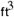Appendix D - Ash Disposal and Grave Volumes

## Appendix D - Ash Disposal and Burial Pits (Continued)

Estimating the Capacities of the Burial Pits: Human Body Proportions from DaVinci

To determine the number of victims that could be buried in a grave of given dimensions, it is first necessary to estimate the volume displaced by a cadaver. Since the people murdered were both male and female, child and adult, the determination is practically limited to a first order approximation of some ideal standard. Here we have chosen an adult male. Using this metric, the number of bodies which can be accommodated by a pit will be a conservative estimate because it will omit smaller stature women and the even smaller children that were killed and interred with their parents.

The voids between bodies cannot be calculated, but the method devised to estimate the volume of an individual body has to be larger than actual volume. This is because the approximation quantitizes the body parts into boxes the size of a man's head, which includes empty space. The proportions of the body come from DaVinci's drawing of the so called Vetruvian Man (see Figure D9). Our adaptation is shown below. The magnitude of w, h , and d were visually estimated in each cell in a row of station(n). The results were then summed for all cells. Table D1 presents the results. The man's statures was assumed to be 68 inches. DaVinci's proportions are based on the head size, so that each cell is 68/8 = 6.8 inches high, the face width 5.9 inches. For example, the head in the figure has the following dimension: h = 6.8, w = 5.9, d = 1.5 X 5.9 = 8.5 inches. This factors to a volume of 341 cubic inches. Since the figure includes all the voids between the curved surface and the enclosing cube, the estimate is on the high side.

 Station(n) 1 2 3 4 5 6 7 8 height-inches 8.4 8.4 8.4 8.4 8.4 8.4 8.4 8.4 width-inches 5.9 11.8 17.7 17.7 11.8 11.8 5.9 5.9 depth-inches 7.1 5.0 10.3 8.8 8.8 7.4 4.4 3.0 Volume-.20 .29 .89 .76 .50 .42 .13 .09
 Table D1 - First Order Estimation of Ideal Man's Body Volume

The sum of V(n) in the table is 3.3 cubic feet. If one were building a coffin for an individual of this size, and if one made sure the interior were 3' X 2' X 8' cells in size, it would fit him snugly, yet leave a lot of empty space, particularly around the legs, head and neck. Such a coffin would enclose 6.6 cubic feet. The SS were interested in efficient use of the burial pits, so it is clear that they made sure the burial commando packed the bodies optimally. So if we use the metric of 3.3 cubic feet as the displacement of the ideal man, any calculation of a graves capacity using that number will be conservative to begin with, and will be more so if we do not factor the women's and children's smaller bodies.

Mass grave site dimensions are usually given as though they were perfect cubes. In actuality, very large grave sites, like those a Treblinka are more like inverted, truncated pyramids. The deeper the grave, the more it had to be sloped so as to avoid the sides from slumping. This was not likely a safety concern - after all the work Jews who had to fill them or to clamber down inside to arrange the bodies were disposable. The SS would have been concerned that such slumping would make for premature filling and boundary irregularities. At Treblinka the soil was very sandy. This fact made for ease of excavation but troublesome stability. Therefore, one can be certain that the sides of each pit were sloped. It is doubtful that there were soil engineering studies done, so the slope angle must have been a guess on the part of the excavator operators, bolstered by empirical experience as they dug along. Viernik cites a depth of ten meters for the pits. If it is accurate, there is even more reason to believe that the sides had to be sloped. How much they were sloped is a pure guess. Soil engineering textbooks have formulas one can plug into to determine the optimum angle, but they require that the soil mechanics be known and can be used as inputs to the equations. Therefore, we have guessed that at Treblinka, if the pits were dug 10 meters deep, the slope angles were 60 degrees. A pit 50 X 25 X 10 meters would look like that in Figure D10.

Due to the sloping sides, the bottom of the pit would be only 13.45 by 38.45 meters. The total volume would be 8502 cubic meters, or 300,000 cubic feet. Filled to the brim, the grave would hold about N bodies the size of the ideal man:

 N = 300,000/3.3 = 91,000
.

In the interest of bounding the problem, note that if all the men were buried in coffins, like the one cited above, N would have an absolute lower bound of 45,500.

It is concluded that a reasonable estimate for the contents of a mass grave 50 X 25 X 10 meters is at least 100,000 people.